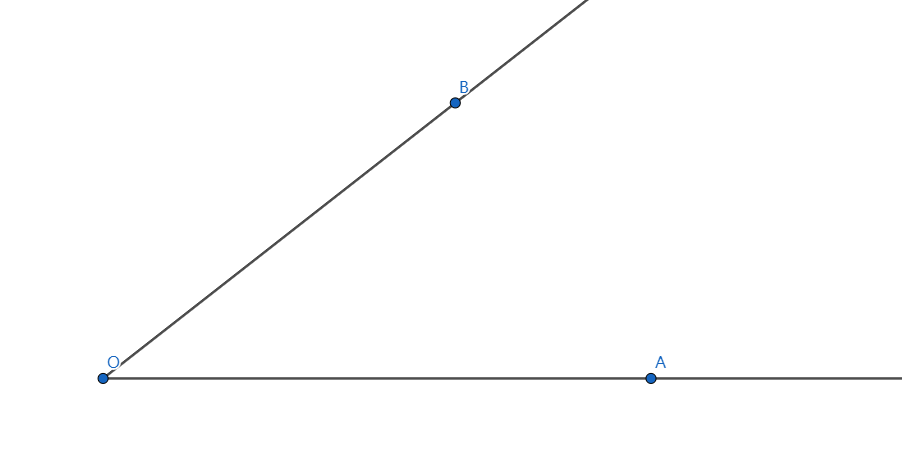# Reach for the Summit - P-S1-A1A UFO is moving at a constant velocity $v$ from $O$ along ray $OB$, and $OA$ is horizontal, as shown in the picture. Alice is observing it at point $A$, and $\angle BOA = \theta$, which can be considered constant during the observation.

Given that the UFO is giving off a small pulse of sound wave and then a big pulse after $\tau\ (\tau \rightarrow 0)$ seconds.

If $OA=L$, and the speed of sound in the air is $v'$, at what condition for $v$ can Alice record the big pulse first and then the small pulse of sound wave?

Take $\theta = 30 \degree$, and the condition is $v > \lambda v'$. Submit $\lfloor 1000\lambda \rfloor$.

Reach for the Summit problem set - Physics

×